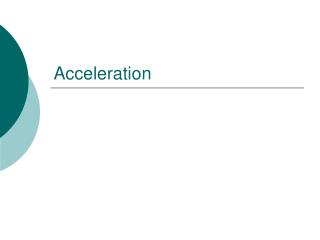DownloadDownload PresentationAcceleration

# Acceleration

Télécharger la présentation## Acceleration

- - - - - - - - - - - - - - - - - - - - - - - - - - - E N D - - - - - - - - - - - - - - - - - - - - - - - - - - -
##### Presentation Transcript

1. Acceleration

2. Understanding Speed vs. Velocity • Speed is simply how fast • Velocity is how fast in a direction • Speed = distance/time • Velocity = displacement/time

3. Self check • The speedometer of a car moving east reads 100 km/h. It passes another car moving west at 100 km/h. Do they have same speed? Velocity? • Same speed but different directions so their velocities are different. • During a certain period of time, the speedometer of a car reads a constant 60 km/h. Does this indicate a constant speed? Constant velocity? • Speed is constant but direction may not be constant so velocity may or may not be constant.

4. Velocity • speed in a given direction. • Velocities in the same direction: (+) Add • + = • 2m/s E + 1m/s E = 3m/s E • Velocities in different directions: (-) Subtract • + = • 2m/s E - 1m/s W = 1m/s E • Examples • Escalator • Treadmill

5. Don’t be so negative • It is important Velocities have direction • Since we can have many different directions, in physics we usually use a + and – sign • Usually this corresponds to the graphing axis (x and y)

6. Acceleration • Acceleration - the rate of change in velocity. • Any time Velocity (Speed or direction) changes • acceleration = v final - v initial ------------- time We can usually feel Accelerations

7. Try It! • If a rocket blasts off at 200km/s N into space and after 8 sec. reaches a velocity of 550km/s N. What was the rocket’s acceleration after take off? • Step 1  acceleration = v final – v initial time • Step 2  acceleration = 550km/s – 200km/s 8 s • Step 3  acceleration = 43.8km/s/s or km/s2

8. Deceleration • Deceleration – a decrease in velocity over time. • Same formula, just different name Deceleration is negative acceleration.

9. Try It! • If a car traveling at a velocity of 50 m/s and quickly stops in 3s for a red light, find the deceleration of the car? • Step 1  deceleration = v final – v initial time • Step 2  deceleration = 0m/s – 50m/s 3 s • Step 3  deceleration = -16.7m/s/s or m/s2

10. Accelerating a Car • Gas Pedal • If acceleration is positive, then velocity is increasing • Brake Pedal • If acceleration is negative, then velocity is decreasing (deceleration) • What other way is there to accelerate your car?

11. Acceleration is indicated by a curve on a Distance-Time graph. Changing slope = changing velocity Distance-Time Graph Graphing Acceleration

12. Graphing Acceleration • A Distance vs. Time Graph • Acceleration is represented by a curved line. • A Speed vs. Time Graph • Acceleration is represented by a straight diagonal line.

13. Graphing Accelerated Motion slope = straight line = flat line = Speed-Time Graph acceleration • + = speeds up • - = slows down constant accel. no accel. (constant velocity)

14. Specify the time period when the object was... slowing down 5 to 10 seconds speeding up 0 to 3 seconds moving at a constant speed 3 to 5 seconds not moving 0 & 10 seconds Speed-Time Graph

15. Warm-Up • What is acceleration? • What is the shape of a distance-time graph for accelerated motion? • What is the shape of a Speed-time graph for accelerated motion? • Why are they different?

16. Momentum

17. Momentum • Momentum – is equal to the mass of an object multiplied by its velocity. • How hard it is to stop a moving object • All moving objects have momentum. http://econtent.bucksiu.org/?a=47768&ch=2

18. http://econtent.bucksiu.org/?a=47768&s=00:01:40:17&e=00:02:30:26http://econtent.bucksiu.org/?a=47768&s=00:01:40:17&e=00:02:30:26 Momentum “Inertia in Motion” Momentum of an object is, (Momentum) = (Mass) X (Velocity) P = M V or M V = P Examples of objects with a large momentum are supertanker (large mass) and bullet (large velocity). http://econtent.bucksiu.org/?a=47768&s=00:04:58:00&e=00:06:07:00

19. Check Yourself A 2 ton car, going 60 m.p.h. hits a 5 ton truck, going 20 m.p.h.. Which vehicle, the car or the truck, has greater momentum? The car because (2)x(60) = 120 and (5)x(20) = 100. What would the car’s speed have to be for the momentums to match? Fifty m.p.h. since (2)x(50) = (5)X(20). Aren’t you forgetting something? The direction of velocity. How does that matter? Rear-end crash not same as a head-on crash. More about this when we do collisions.

20. Conservation of Momentum • Law of Conservation of Momentum – states that the total momentum of any group of objects remains the same unless outside forces act on the object. http://econtent.bucksiu.org/?a=47768&s=00:07:10:28&e=00:08:01:00

21. Recoil Momentum conservation also explains recoil (MASS) x (velocity) (mass) x (VELOCITY)

22. Wrap Up! • What is momentum? • What is the law of conservation of momentum? • Using the formula for calculating momentum, determine the units of measurement?